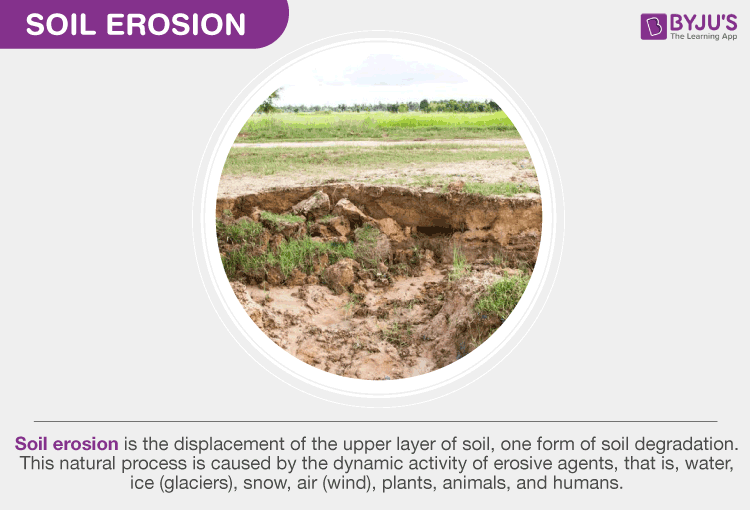# Soil Erosion FormulaThe loss of soil mass over time for a specific landmass is known as soil erosion. It affects farming, construction projects, and house owners living near oceans, rivers, and terrestrial slopes. To predict the soil erosion due to water, scientists developed a formula known as the Universal Soil loss equation. The soil erosion formula foretells the long-term average annual rate of erosion on a field slope based on soil type, rainfall pattern, topography, management practices, and crop system. In the article, let us discuss the soil erosion formula.

## Universal Soil Loss Equation

The universal soil loss equation predicts the annual soil loss per unit area. The universal soil loss equation is given by

$$\begin{array}{l}A=R\times K\times L\times S\times CP\end{array}$$

Here,

• A is the estimated annual soil loss
• R is the rainfall and runoff factor representing the summed erosive potential of all rainfall events in a year.
• L is the slope length
• S is the slope steepness
• K is the soil erodibility factor representing units of soil loss per unit of soil erosivity
• CP characterizes conservation management and land cover practices.

### Using the Soil Erosion Formula

To use the universal soil equation, one needs to obtain the value of the R factor from the local station. Determine the kind of soil and assign a value for the K-factor. Measure the length of the slope of the land and identify the percentage of the slope to derive the LS factor. If the land is not used for crops, then the C and P factors are generally equal to one. These factors vary between zero and one if the land is actively farmed and tilled.

Stay tuned to BYJU’S to learn more formula of various physics concepts such as the Maxwell Boltzmann distribution formula.